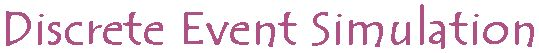## Lesson 1

### Introduction to Discrete Event Simulation

In this lesson we will introduce you to some basic concepts of Discrete Event Simulation:

• D.E.S = Discrete Event Systems
• Virtual time
• Event
• System state
• F.E.S = Future Event Set
Also we have developed two examples showing some simple simulations:

Simulation means using a model that mimics a real world system, analyzing model output obtained with suitable input, in order to observe the behaviour of the real world system. A model is a description of some system intended to predict what happens if certain actions are taken. All useful models simplify and idealize real world systems. One way to simplify a real world system is by using discrete event models.

Discrete event simulation regards a real world system as a set of states. For instance, when simulating a queue in front of an elevator one of the system states represents the number of people in the queue. Another one represents the number of people in the elevator and so on. During the simulation people arrive in front of the elevator making the queue longer, while others step in the elevator making the queue shorter. Thus, the arrivals and departures of people bring forth changes in one of the system states. These changes are called events. When the number of events is finite we call the simulation discrete event.

In a discrete event simulation, the future event set is the data structure that holds the records of the events scheduled to occur in the future, in chronological order. During simulation the first event in the future event set is taken out of the set and all the actions associated with it are performed. Thus, the event is handled. Handling of an event may imply creating other events that will take place in the future and placing these in the future event set. After the current event is handled and taken out of the future event set, the simulation moves on to the next event of the future event set.
For instance, when simulating a queue in front of an elevator, every arrival of a person that steps into a queue is an event. Usually the simulation determines the arrival times of people and puts them in the future event set. When the simulation starts it steps to the first such arrival of a person and handles it. If for instance the person steps in the elevator and activates it, the return-time of the elevator is calculated and this event is put in the future event set. After that the simulation continues on to the next event, which may either be another arrival of a person, or the arrival of the elevator. When there are no more events in the future event set, the simulation stops.

When one uses a discrete event simulation one has to keep in mind that there are two different types of time involved. The first type is called CPU-time, denoting the time in which the simulation has been running on the computer. In fact, this is the time as we experience it in the real world. The second type is called virtual time, denoting the time as it elapses inside the simulation model.
For instance, one is interested of the average waiting time in a queue in front of an elevator. So one could observe the queue for the duration of a week. To save some time, one can also create a simulation which will simulate the queue for the duration of a week, but which takes only a few seconds to run. The system-time is now only a few seconds, while the virtual time is one week.

Some of these concepts may be understood better by viewing the following example simulations: